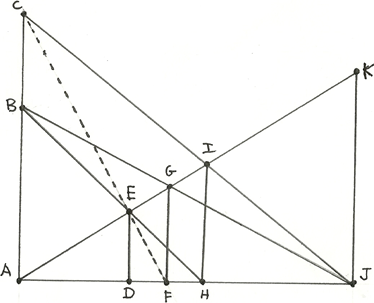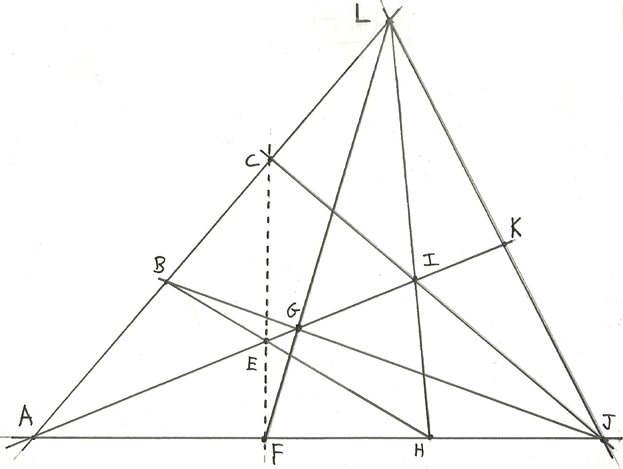## Thursday, October 31, 2013

### On Reduction, 9 of 11

Reciprocal Calculus

Define a function’s “protractional fluxion”, or “pluxion”, this way:

Pf   =  pf/px   =   lim  (f(x) <-> f(y)) / (x <-> y)
y->x

=   lim  (f(x<+>H) <-> f(x)) /  H
H->∞

I think Newton’s term “fluxion” is a fine one, and it deserves more use; though here it is an abbreviation for “differential fluxion”. Where the differential fluxion is the ratio of two infinitesimal differences, the protractional fluxion is the ratio of two infinite protractions. Since the algebraic laws of reduction mirror those of addition, we can derive the following pluxion laws in the usual ways:

Px      =   1

PK      =              for any constant K

P( K f)   =   K P(f)      for any constant K

P(f<+>g)  =   P(f) <+> P(g)

P(f<->g)  =   P(f) <-> P(g)

P(f*g)  =   P(f)*g  <+>  f*P(g)

P(1/g)  =   - P(g)/g2

P(f/g)  =   ( P(f)*g  <-> f*P(g) ) / g2

P(x2)   =   x/2

P(x3)   =   x2/3

And in general;       P(xN)   =   xN-1/N

So we can protractionate reductive polynomials. For instance:

P( 7 x3 <+> 5x2 <-> 3x <+> 11 )  =  (7/3)x2 <+> (5/2)x <-> 3

Pluxions have the chain rule:

pz/px       =      pz/py    *  py/px

P(f(g(x)))   =  ((Pf)(g(x))) * (Pg)(x)

Recall the Complementarity rule;

(a-b)(a<->b) = -ab;

protraction times difference equals negative product.

Apply this to the product of pluxion and fluxion:

For y near to x, (pf/px)*(df/dx) approximately equals:

{(f(x)<->f(y))/ (x<->y) }*{(f(x)-f(y))/ (x-y) }

=    {(f(x)<->f(y))*(f(x)-f(y))} / {(x<->y)*(x-y)}

=    { - f(x) * f(y) } / { - x * y }

Which in the limit approaches

(f(x))2 / x2

Therefore in general:

(pf/px)*(df/dx)  =   (f(x))2 / x2

Pf * f’         =    f2/x2

Therefore the Pluxion from Fluxion law:

pf/px   =    (f(x))2 / ( x2 * df/dx )

Pf      =     f2 /(x2 . f’)

In particular:

P(ex)         =     ex /x2

P(e-1/x)       =     e-1/x

P(ln(x))      =    (ln(x))2 / x

P(-1/ln(x))   =     1 / x

We can now derive P(f+g):

P(f+g)     =    (f+g)2 / (x2(f+g)’)

=    (f+g)2 / (x2(f’+g’))

=    (f+g)2( 1/(x2f’)  <+>  1/(x2g’) )

=    (f+g)2  ( Pf/f2  <+>  Pg/g2 )

Similarly;

(f<+>g)’   =    (f<+>g)2  ( f’/f2  +  g’/g2 )

For instance;

(x<+>x2)’  =    (x<+>x2)2  ( x’/x2  +  x2’/(x2)2 )

=    x2(1<+>x)2 ( 1/x2   +  2x/x4 )

=    (x2*x2/(x+1)2)( 1/x2   +  2/x3 )

=    (x4/(x+1)2)( (x + 2) / x3 )

=    x(x+2)/(x+1)2

P(x+x2)    =    (x+x2)2  ( Px/x2  <+>  Px2/(x2)2 )

=    x2(1+x)2 ( 1/x2   <+>  (x/2)/x4 )

=    x2(1+x)2 ( 1/x2   <+>  (1/2x3 )

=    (1+x)2 ( 1  <+>  (1/2x) )

=    (1+x)2 ( 1 / (1+2x) )

=    (1+x)2 / (1+2x)

For longer sums we have:

P(f+g+h)   =    (f+g+h)2  ( Pf/f2  <+>  Pg/g2  <+>  Ph/h2)

(f<+>g<+>h)’    =    (f<+>g<+>h)2  ( f’/f2  +  g’/g2  +  h’/h2)

And so on.

One of the uses of the fluxion is in Newton’s Method for finding the roots of a function. To solve f(x) = 0, start with an estimate x1, then run this iteration:

XN+1   =   XN – f(XN)/f’(XN)

But   Pf   =   f2 /(x2 . f’)

so   f/f’ =    x2 Pf / f

and therefore

XN+1   =   XN  XN2 P(XN)/f(XN)

XN+1   =   XN (  1    XN*Pf(XN)/f(XN) )

This is the pluxion version of Newton’s Method.

Another use of the fluxion is finding extrema. But since Pf*f’ = f2/x2, f’>0 only if Pf>0; f’=0 only if Pf=∞; and f’<0 only if Pf<0. So you may find critical points by solving  Pf(x)= ∞;  then examine Pf near the critical point to tell if it is an extremum or not. If Pf goes from – through ∞ to +, then the critical point is a minimum; if Pf goes from + through ∞ to – then the critical point is a maximum. This is the First Pluxion Test.

Inverse to the Pluxion is the Indefinite Reciprocal Integral, also called the Anti-pluxion:

R( f(x) px )  =  F(x) <+> C   if and only if   P(F(x)) = f(x)

C is the constant of reciprocal integration.

There’s also a Definite Reciprocal Integral:

Rab(f(x) px)  =

lim  ( f(a)<+>f(a<+>H)<+>f(a<+>H/2)<+>f(a<+>H/3)<+><+>f(b) )* H )
H->∞

These two are united by the Fundamental Theorem of Reciprocal Calculus:

If    F = Rax(f(y) py)    then     pF/px = f

If   pF/px  =  f    then    Rab(f(x) px) =  F(b) y F(a)

Here is a short table of reciprocal integrals:

R( 1 px )      =      x

R( ∞ px )      =      C  ,  a constant.

R(K*f px )      =     K * R(f px)    ,   for any constant K.

R((f <+> g)px )   =        R(f px) <+> R(g px)

R((f <-> g)px )   =        R(f px) <-> R(g px)

R( xN px )      =      (N+1) xN+1

R( (1/x) px )   =     -1 / ln(x)   =  logx(1/e)

R( e-1/x  px )   =       e-1/x

Here’s the Chain Rule:

R( f(g(x)) Pg(x) px )   =   (Rf)(g(x))

Here’s Reductive Integration by Parts:

R( Pf * g * px )    =   f*g  <->  R( f * Pg * px )

R ( g * pf )        =   f*g  <->  R( f * pg )

## Wednesday, October 30, 2013

### On Reduction, 8 of 11

Projective Reduction

Recall the “fish-tail” diagram:

*     *
|\   /|
| \ / |
x |  *  | y        z  =  x<+>y
| /|\ |
|/ |z\|
*--*--*

If you flip this diagram left for right, then the lengths x, y and z remain the same; so x<+>y = y<+>x. Commutativity is illustrated by reflection.
If you stretch it vertically then x, y and z become kx, ky and kz; so k(x<+>y)  =  kx <+> ky. So distribution is illustrated by stretching.
So commutativity and distribution for reduction relate to the symmetries of the fish-tail diagram. The associative law is more complicated. It is illustrated by this diagram:AC, DE, FG, HI and JK are parallel;
ABC, CIJ, BGJ, BEH, AEGIK and ADFHJ are collinear.

If AC = x, JK = y and AB = z, then
IH = x<+>y  ;  GF = y<+>z  ;  DE = (x<+>y) <+>z
But (x<+>y)<+>z  = x<+>(y<+>z);
So the AC and FG fishtail meets at E;
Therefore C, E and F are collinear.
If AC, DE, FG, IH and JK are parallel;
and ABC, CIJ, BGJ, BEH, AEGIK and ADFHJ are collinear;
then CEF are collinear.

That theorem in affine geometry is equivalent to the associative law for reduction. I therefore call this the Affine Reductive Associative Theorem. Here is a projective version of the diagram, with D omitted, and including L as a point at infinity:Given these collinearities:
ABCL, FGL, HIL, JKL, CIJ, BGJ, BEH, AEGIK, AFHJ
Then CEF are collinear.

This theorem is a consequence of Pappus’s Hexagon Theorem: for BCL are collinear points and FHJ are collinear points; therefore the opposite sides of the hexagon BHLFCJ meet in three collinear points: EGI. This proof from Pappus does not assume that the three lines BCL, FHJ and EGI meet at a point, as they do here.
So the associativity of reduction is a consequence of a special case of Pappus’s Hexagon Theorem!